# 使用云存储服务来进行终端之间的数据交换

3 十月 2017, 08:24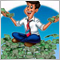0
1 960

### 1. 一点历史

Google Drive（谷歌云盘） 的认证是通过 OAuth 2.0 协议的，这是一项开放的认证协议，第三方应用程序和网站可以对认证用户保护的资源进行有限的访问而不需要传输密码信息。基本的 OAuth 2.0 访问场景包含4步，

1. 首先，您需要取得用于认证的数据 (客户的 ID 和密码)，这些数据是由网站生成的，并且网站和应用程序都是知道的。
2. 在应用程序能够访问个人数据之前，它应当收到访问令牌，一个这样的令牌可以提供由'scope'变量定义的不同级别的访问。当请求访问令牌时，应用程序可以在'scope'参数中发送一个或多个值，为了创建这个请求，应用程序可以使用系统浏览器和 web service 请求。有些请求需要在用户登录他们账户的时候有一个认证步骤，在登录之后，用户被询问，他们是否准备给应用程序请求以许可，这个过程被称为用户同意(user consent)。如果用户提出同意，认证服务器就给应用程序提供认证编码，使得应用程序可以获得访问令牌，如果用户没有同意，服务器就返回错误。
4. 令牌的生命周期是有限的，如果应用程序在访问令牌过期后还需要访问，它可以取得一个更新令牌来允许应用程序取得新的访问令牌。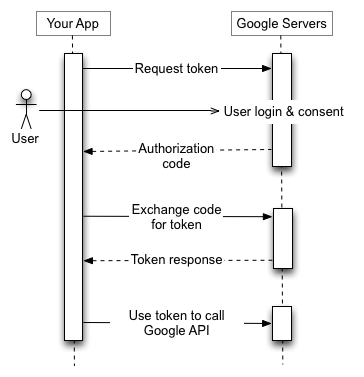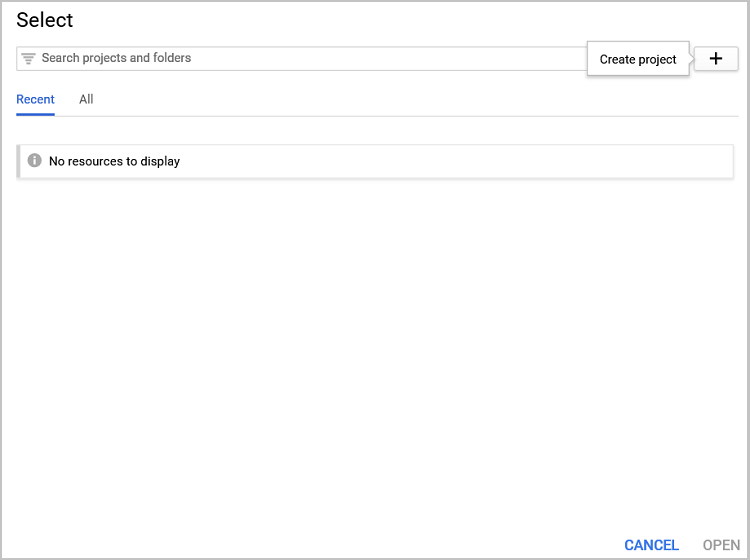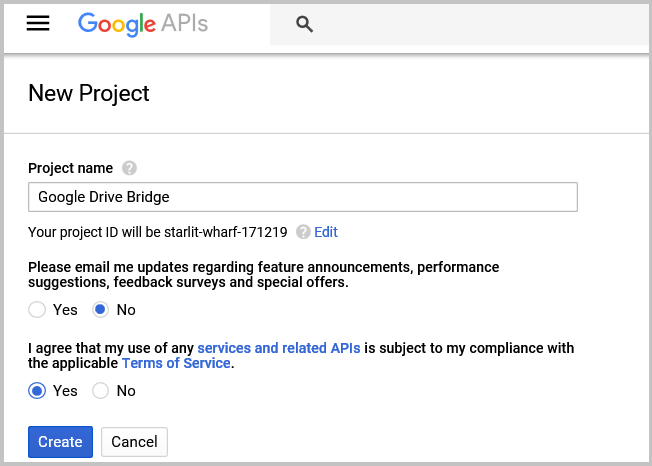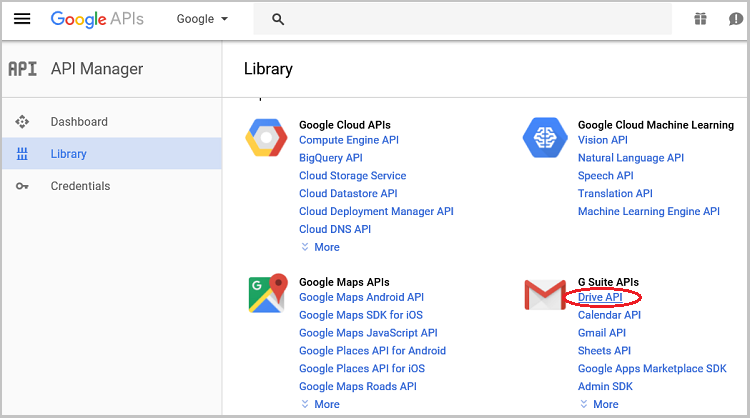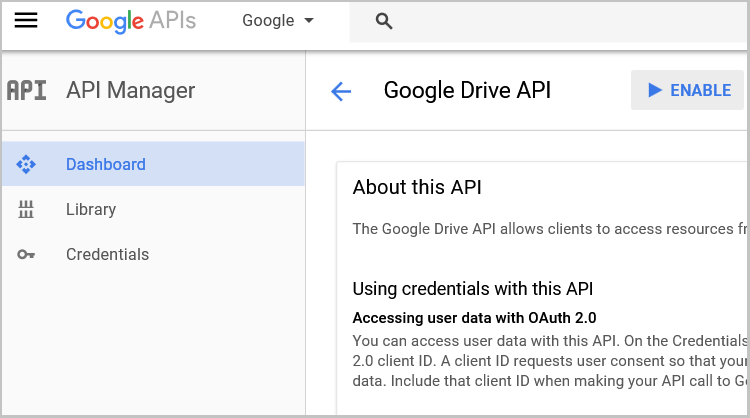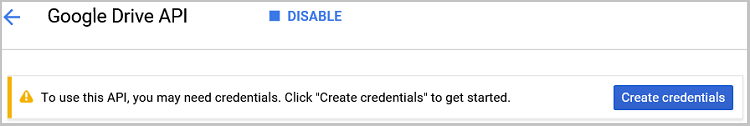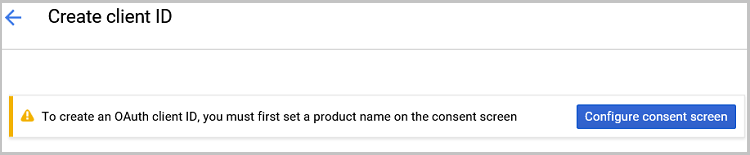### 3. 在本地应用程序和 Google Drive 之间创建桥梁

```class GoogleDriveClass
{
static string[] Scopes = { DriveService.Scope.DriveFile };  //用于操作类的数组
static string ApplicationName = "Google Drive Bridge";      //应用程序名称
public static UserCredential credential = null;             //认证密钥
public static string extension = ".gdb";                    //所保存文件的扩展名
}
```

```        public bool Authorize()
{
using (System.IO.FileStream stream =
{
try
{
string credPath = System.Environment.CurrentDirectory.ToString();
credPath = System.IO.Path.Combine(credPath, "drive-bridge.json");

"example.bridge@gmail.com",
CancellationToken.None,
new FileDataStore(credPath, true)).Result;
}
catch (Exception)
{
credential = null;
}

}
return (credential != null);
}

```

```using File = Google.Apis.Drive.v3.Data.File;
public IList<File> GetFileList()
{
IList<File> result = null;
if (credential == null)
this.Authorize();
if (credential == null)
{
return result;
}
// 创建 Drive API 服务.
{
HttpClientInitializer = credential,
ApplicationName = ApplicationName,
}))
{
try
{
// 定义请求的参数.
FilesResource.ListRequest listRequest = service.Files.List();
listRequest.PageSize = 1000;
listRequest.Fields = "nextPageToken, files(id, name, size)";

// 列出文件.
result = listRequest.Execute().Files;
}
catch (Exception)
{
return null;
}
}
return result;
}

```

#### 3.1. 想云存储中写入数据

```       public bool FileCreate(string name, string value, out string id)
{
bool result = false;
id = null;
if (credential == null)
this.Authorize();
if (credential == null)
{
return result;
}
using (var service = new Google.Apis.Drive.v3.DriveService(new BaseClientService.Initializer()
{
HttpClientInitializer = credential,
ApplicationName = ApplicationName,
}))

{
var body = new File();
body.Name = name;
body.MimeType = "text/json";
body.ViewersCanCopyContent = true;

byte[] byteArray = Encoding.Default.GetBytes(value);
using (var stream = new System.IO.MemoryStream(byteArray))
{
{ id = request.ResponseBody.Id; result = true; }
}
}
return result;
}
```

```        public bool FileUpdate(string name, string value)
{
bool result = false;
if (credential == null)
this.Authorize();
if (credential == null)
{
return result;
}

string new_id;
if (FileCreate(name, value, out new_id))
{
IList<File> files = GetFileList();
if (files != null && files.Count > 0)
{
result = true;
try
{
using (var service = new DriveService(new BaseClientService.Initializer()
{
HttpClientInitializer = credential,
ApplicationName = ApplicationName,
}))
{
foreach (var file in files)
{
if (file.Name == name && file.Id != new_id)
{
try
{
string res = request.Execute();
}
catch (Exception)
{
continue;
}
}
}
}
}
catch (Exception)
{
return result;
}
}
}
return result;
}
```

#### 3.2. 从云存储中读取数据

```        public string GetFileId(string name)
{
string result = null;
IList<File> files = GetFileList();

if (files != null && files.Count > 0)
{
foreach (var file in files)
{
if (file.Name == name)
{
result = file.Id;
break;
}
}
}
return result;
}
```

```        public string FileRead(string id)
{
if (String.IsNullOrEmpty(id))
{
return ("错误. 没有找到文件");
}
bool result = false;
string value = null;
if (credential == null)
this.Authorize();
if (credential != null)
{
using (var service = new DriveService(new BaseClientService.Initializer()
{
HttpClientInitializer = credential,
ApplicationName = ApplicationName,
}))
{
using (var stream = new MemoryStream())
{
{
result = true;
};

if (result)
{
int start = 0;
int count = (int)stream.Length;
value = Encoding.Default.GetString(stream.GetBuffer(), start, count);
}
}
}
}
return value;
}
```

#### 3.3. 使用终端应用程序创建一些交互

• Drive — 之前创建的用于操作云存储的类;
• pipeName — 用于保存我们管道名称的字符串变量;
• servers — 运行线程的数组.
```        GoogleDriveClass Drive = new GoogleDriveClass();
private static int numThreads = 10;
private static string pipeName = "GoogleBridge";

```

```        public void PipesCreate()
{
int i;

for (i = 0; i < numThreads; i++)
{
servers[i].Start();
}
}
```

```        private void ServerThread()
{
NamedPipeServerStream pipeServer =
new NamedPipeServerStream(pipeName, PipeDirection.InOut, numThreads, PipeTransmissionMode.Message, PipeOptions.Asynchronous);

// 等待客户端连接
AsyncCallback asyn_connected = new AsyncCallback(Connected);
try
{
pipeServer.BeginWaitForConnection(asyn_connected, pipeServer);
}
catch (Exception)
{
}
}

```

```        private void Connected(IAsyncResult pipe)
{
if (!pipe.IsCompleted)
return;
bool exit = false;
try
{
NamedPipeServerStream pipeServer = (NamedPipeServerStream)pipe.AsyncState;
try
{
if (!pipeServer.IsConnected)
pipeServer.WaitForConnection();
}
catch (IOException)
{
AsyncCallback asyn_connected = new AsyncCallback(Connected);
pipeServer.Dispose();
pipeServer = new NamedPipeServerStream(pipeName, PipeDirection.InOut, numThreads, PipeTransmissionMode.Message, PipeOptions.Asynchronous);
pipeServer.BeginWaitForConnection(asyn_connected, pipeServer);
return;
}
while (!exit && pipeServer.IsConnected)
{
// 从客户端读取请求. 当客户端
// 写入管道时，它的安全令牌就可用了.

while (pipeServer.IsConnected)
{
{
exit = true;
break;
}
}
//等待一个客户端的连接
AsyncCallback asyn_connected = new AsyncCallback(Connected);
pipeServer.Disconnect();
pipeServer.BeginWaitForConnection(asyn_connected, pipeServer);
break;
}
}
finally
{
exit = true;
}
}
```

• Close — 关闭当前的连接;
• Write — 把文件写到云存储.

```        private bool ReadMessage(PipeStream pipe)
{
if (!pipe.IsConnected)
return false;

string message = null;
int length;
do
{
if (length > 0)
} while (length >= 1024 && pipe.IsConnected);
if (message == null)
return true;

if (message.Trim() == "Close\0")
return false;

string result = null;
string[] separates = { ";" };
string[] arr_message = message.Split(separates, StringSplitOptions.RemoveEmptyEntries);
{
try
{
}
catch (Exception e)
{
result = "错误 " + e.ToString();
Drive.Authorize();
}
return WriteMessage(pipe, result);
}

if (arr_message.Trim() == "Write")
{
try
{
result = (Drive.FileUpdate(arr_message.Trim() + GoogleDriveClass.extension, arr_message.Trim()) ?"完成" : "错误");
}
catch (Exception e)
{
result = "错误 " + e.ToString();
Drive.Authorize();
}

return WriteMessage(pipe, result);
}
return true;
}
```

```        private bool WriteMessage(PipeStream pipe, string message)
{
if (!pipe.IsConnected)
return false;
if (message == null || message.Count() == 0)
message = "Empty";
byte[] arr_bytes = Encoding.Default.GetBytes(message);
try
{
pipe.Flush();
pipe.Write(arr_bytes, 0, arr_bytes.Count());
pipe.Flush();
}
catch (IOException)
{
return false;
}
return true;
}
```

```        public Form1()
{
InitializeComponent();
PipesCreate();
}
```

#### 4.1. 用于操作图形对象的类

```enum ENUM_SET_TYPE
{
ENUM_SET_TYPE_INTEGER=0,
ENUM_SET_TYPE_DOUBLE=1,
ENUM_SET_TYPE_STRING=2
};
```

```class CCopyObject
{
private:
string            s_ObjectsID;

public:
CCopyObject(string objectsID="CopyObjects");
~CCopyObject();
};
//+------------------------------------------------------------------+
//|                                                                  |
//+------------------------------------------------------------------+
CCopyObject::CCopyObject(string objectsID="CopyObjects")
{
s_ObjectsID = (objectsID==NULL || objectsID=="" ? "CopyObjects" : objectsID);
}
```

4.1.1. 用于收集对象数据的函数

```string CCopyObject::CreateMessage(long chart)
{
string result = NULL;
int total = ObjectsTotal(chart, 0);
for(int i=0;i<total;i++)
{
string name = ObjectName(chart, i, 0);
switch((ENUM_OBJECT)ObjectGetInteger(chart,name,OBJPROP_TYPE))
{
case OBJ_HLINE:
result+="{NAME="+name+"|TYPE="+IntegerToString(OBJ_HLINE)+"|"+HLineToString(chart, name)+"}";
break;
case OBJ_VLINE:
result+="{NAME="+name+"|TYPE="+IntegerToString(OBJ_VLINE)+"|"+VLineToString(chart, name)+"}";
break;
case OBJ_TREND:
result+="{NAME="+name+"|TYPE="+IntegerToString(OBJ_TREND)+"|"+TrendToString(chart, name)+"}";
break;
case OBJ_RECTANGLE:
result+="{NAME="+name+"|TYPE="+IntegerToString(OBJ_RECTANGLE)+"|"+RectangleToString(chart, name)+"}";
break;
}
}
return result;
}
```

```string CCopyObject::HLineToString(long chart,string name)
{
string result = NULL;
if(ObjectFind(chart,name)!=0)
return result;

result+=IntegerToString(ENUM_SET_TYPE_DOUBLE)+"="+IntegerToString(OBJPROP_PRICE)+"=0="+DoubleToString(ObjectGetDouble(chart,name,OBJPROP_PRICE,0))+"|";
result+=IntegerToString(ENUM_SET_TYPE_INTEGER)+"="+IntegerToString(OBJPROP_COLOR)+"=0="+IntegerToString(ObjectGetInteger(chart,name,OBJPROP_COLOR,0))+"|";
result+=IntegerToString(ENUM_SET_TYPE_INTEGER)+"="+IntegerToString(OBJPROP_STYLE)+"=0="+IntegerToString(ObjectGetInteger(chart,name,OBJPROP_STYLE,0))+"|";
result+=IntegerToString(ENUM_SET_TYPE_INTEGER)+"="+IntegerToString(OBJPROP_BACK)+"=0="+IntegerToString(ObjectGetInteger(chart,name,OBJPROP_BACK,0))+"|";
result+=IntegerToString(ENUM_SET_TYPE_INTEGER)+"="+IntegerToString(OBJPROP_WIDTH)+"=0="+IntegerToString(ObjectGetInteger(chart,name,OBJPROP_WIDTH,0))+"|";
result+=IntegerToString(ENUM_SET_TYPE_STRING)+"="+IntegerToString(OBJPROP_TEXT)+"=0="+ObjectGetString(chart,name,OBJPROP_TEXT,0)+"|";
result+=IntegerToString(ENUM_SET_TYPE_STRING)+"="+IntegerToString(OBJPROP_TOOLTIP)+"=0="+ObjectGetString(chart,name,OBJPROP_TOOLTIP,0);
return result;
}
```

4.1.2. 用于在图表上绘制对象的函数

• 把字符串消息根据对象分成字符串数组;
• 把每个对象数组元素分成参数的数组;
• 在参数数组中寻找名称和对象类型. 把我们的ID加到名称中;
• 根据获得的名称在图表上寻找对象；如果对象不在主窗口或者它的类型与消息中指定的不同，就删除它;
• 如果还没有对象或者在前一步中被删除，就在图表上创建一个新的对象;
• 把消息中接收到的对象属性传输到我们图表上的对象中(使用另外的 CopySettingsToObject 函数);
• 从图表上删除不需要的对象 (由另外的 DeleteExtraObjects 函数进行)。
```bool CCopyObject::DrawObjects(long chart,string message)
{
//--- 把消息分割成对象
StringTrimLeft(message);
StringTrimRight(message);
if(message==NULL || StringLen(message)<=0)
return false;
StringReplace(message,"{","");
string objects[];
if(StringSplit(message,'}',objects)<=0)
return false;
int total=ArraySize(objects);
SObject Objects[];
if(ArrayResize(Objects,total)<0)
return false;

//--- 把每个对象消息分割成设置
for(int i=0;i<total;i++)
{
string settings[];
int total_settings=StringSplit(objects[i],'|',settings);
//--- 搜索名称和对象的类型
int set=0;
while(set<total_settings && Objects[i].name==NULL && Objects[i].type==-1)
{
string param[];
if(StringSplit(settings[set],'=',param)<=1)
{
set++;
continue;
}
string temp=param;
StringTrimLeft(temp);
StringTrimRight(temp);
if(temp=="NAME")
{
Objects[i].name=param;
StringTrimLeft(Objects[i].name);
StringTrimRight(Objects[i].name);
Objects[i].name=s_ObjectsID+Objects[i].name;
}
if(temp=="TYPE")
Objects[i].type=(int)StringToInteger(param);
set++;
}
//--- 如果名称或者对象类型没有找到，就转到下一个对象
if(Objects[i].name==NULL || Objects[i].type==-1)
continue;
//--- 在图表上搜索对象
int subwindow=ObjectFind(chart,Objects[i].name);
//--- 如果在图表上找到了对象，但是它不在主窗口或者它的类型不同，我们就从图表上删除这个对象
if(subwindow>0 || (subwindow==0 && ObjectGetInteger(chart,Objects[i].name,OBJPROP_TYPE)!=Objects[i].type))
{
if(!ObjectDelete(chart,Objects[i].name))
continue;
subwindow=-1;
}
//--- 如果对象没有找到，就在图表上创建它
if(subwindow<0)
{
if(!ObjectCreate(chart,Objects[i].name,(ENUM_OBJECT)Objects[i].type,0,0,0))
continue;
ObjectSetInteger(chart,Objects[i].name,OBJPROP_HIDDEN,true);
ObjectSetInteger(chart,Objects[i].name,OBJPROP_SELECTABLE,false);
ObjectSetInteger(chart,Objects[i].name,OBJPROP_SELECTED,false);
}
//---
CopySettingsToObject(chart,Objects[i].name,settings);
}
//---
DeleteExtraObjects(chart,Objects);
return true;
}
```

```bool CCopyObject::CopySettingsToObject(long chart,string name,string &settings[])
{
int total_settings=ArraySize(settings);
if(total_settings<=0)
return false;

for(int i=0;i<total_settings;i++)
{
string setting[];
int total=StringSplit(settings[i],'=',setting);
if(total<3)
continue;
switch((ENUM_SET_TYPE)StringToInteger(setting))
{
case ENUM_SET_TYPE_INTEGER:
ObjectSetInteger(chart,name,(ENUM_OBJECT_PROPERTY_INTEGER)StringToInteger(setting),(int)(total==3 ? 0 : StringToInteger(setting)),StringToInteger(setting[total-1]));
break;
case ENUM_SET_TYPE_DOUBLE:
ObjectSetDouble(chart,name,(ENUM_OBJECT_PROPERTY_DOUBLE)StringToInteger(setting),(int)(total==3 ? 0 : StringToInteger(setting)),StringToDouble(setting[total-1]));
break;
case ENUM_SET_TYPE_STRING:
ObjectSetString(chart,name,(ENUM_OBJECT_PROPERTY_STRING)StringToInteger(setting),(int)(total==3 ? 0 : StringToInteger(setting)),setting[total-1]);
break;
}
}
return true;
}
```

```void CCopyObject::DeleteExtraObjects(long chart,SObject &Objects[])
{
int total=ArraySize(Objects);
for(int i=0;i<ObjectsTotal(chart,0);i++)
{
string name=ObjectName(chart,i,0);
if(StringFind(name,s_ObjectsID)!=0)
continue;
bool found=false;
for(int obj=0;(obj<total && !found);obj++)
{
if(name==Objects[obj].name && ObjectGetInteger(chart,name,OBJPROP_TYPE)==Objects[obj].type)
{
found=true;
break;
}
}
if(!found)
{
if(ObjectDelete(chart,name))
i--;
}
}
return;
}
```

#### 4.2. 提供者应用程序

`sinput bool       SendAtStart =  true; //在 Init 中发送数据`

```#include <CopyObject.mqh>
#include <Files\FilePipe.mqh>

```

```CCopyObject *CopyObjects;
string PrevMessage;
uchar Close[];
```

```int OnInit()
{
//---
CopyObjects = new CCopyObject();
PrevMessage="Init";
StringToCharArray(("Close"),Close,0,WHOLE_ARRAY,CP_UTF8);
if(SendAtStart)
SendMessage(ChartID());
//---
return(INIT_SUCCEEDED);
}
```

```void OnDeinit(const int reason)
{
//---
if(CheckPointer(CopyObjects)!=POINTER_INVALID)
delete CopyObjects;
}
```

```void OnChartEvent(const int id,
const long &lparam,
const double &dparam,
const string &sparam)
{
//---
int count=10;
switch(id)
{
case CHARTEVENT_OBJECT_CREATE:
case CHARTEVENT_OBJECT_DELETE:
case CHARTEVENT_OBJECT_CHANGE:
case CHARTEVENT_OBJECT_DRAG:
case CHARTEVENT_OBJECT_ENDEDIT:
while(!SendMessage(ChartID()) && !IsStopped() && count>=0)
{
count--;
Sleep(500);
}
break;
}
}
```

• 检查用于操作图形对象类的状态，如有必要重新初始化它;
• 使用之前创建的CreatMessage函数来生成要发送到云存储的消息，如果消息为空或者等于上一次发送的，就退出函数;
• 根据图表交易品种生成要发送到云端的文件名称;
• 与我们的桥梁应用程序使用命名管道建立连接;
• 传递命令通过打开的连接把消息以指定文件名发送到云存储;
• 在接收到执行命令的回应之后，发送命令关闭到云端的连接并中断与桥梁应用程序的命名管道;
• 在退出应用程序之前删除用于操作命名管道的对象。

```bool SendMessage(long chart)
{
Comment("发送消息");
if(CheckPointer(CopyObjects)==POINTER_INVALID)
{
CopyObjects = new CCopyObject();
if(CheckPointer(CopyObjects)==POINTER_INVALID)
return false;
}
string message=CopyObjects.CreateMessage(chart);
if(message==NULL || PrevMessage==message)
return true;

string Name=SymbolInfoString(ChartSymbol(chart),SYMBOL_CURRENCY_BASE)+SymbolInfoString(ChartSymbol(chart),SYMBOL_CURRENCY_PROFIT);
CFilePipe *pipe=new CFilePipe();
if(handle<=0)
{
Comment("没有找到管道");
delete pipe;
return false;
}
uchar iBuffer[];
int size=StringToCharArray(("Write;"+Name+";"+message),iBuffer,0,WHOLE_ARRAY,CP_UTF8);
if(pipe.WriteArray(iBuffer)<=0)
{
Comment("发送请求失败");
pipe.Close();
delete pipe;
return false;
}
ArrayFree(iBuffer);
uint res=0;
do
{
}
while(res==0 && !IsStopped());

if(res>0)
{
string result=CharArrayToString(iBuffer,0,WHOLE_ARRAY,CP_UTF8);
if(result!="Ok")
{
Comment(result);
pipe.WriteArray(Close);
pipe.Close();
delete pipe;
return false;
}
}
PrevMessage=message;
pipe.WriteArray(Close);
pipe.Close();
delete pipe;
Comment("");
return true;
}
```

#### 4.3. 用户应用程序

```#include <CopyObject.mqh>
#include <Files\FilePipe.mqh>

```

```sinput int        RefreshTime =  10; //刷新数据的时间，秒数
sinput bool       DeleteAtClose = true;   //在关闭程序时从图表上删除对象
```

```CCopyObject *CopyObjects;
string PrevMessage;
bool timer;
uchar Close[];
```

OnInit 函数中，初始化全局变量和计时器。

```int OnInit()
{
//---
CopyObjects = new CCopyObject(ObjectsID);
PrevMessage="Init";
timer=EventSetTimer(1);
if(!timer)
{
Comment("设置计时器错误");
}
LastRefresh=0;
StringToCharArray(("Close"),Close,0,WHOLE_ARRAY,CP_UTF8);

//---
return(INIT_SUCCEEDED);
}
```

OnDeinit 去初始化函数中，删除用于操作图形对象的类的对象，停止计时器，清除注释并且（如有必要）从图表中删除由应用程序创建的对象。

```void OnDeinit(const int reason)
{
//---
if(CheckPointer(CopyObjects)!=POINTER_INVALID)
delete CopyObjects;
EventKillTimer();
Comment("");
if(DeleteAtClose)
{
for(int i=0;i<ObjectsTotal(0,0);i++)
{
string name=ObjectName(0,i,0);
if(StringFind(name,ObjectsID,0)==0)
{
if(ObjectDelete(0,name))
i--;
}
}
}
}
```

OnTick 函数中，检查计时器状态，并且如果必要重新激活它。

```void OnTick()
{
//---
if(!timer)
{
timer=EventSetTimer(1);
if(!timer)
{
Comment("设置计时器错误");
}
OnTimer();
}
}
```

```void OnTimer()
{
//---
{
Comment("");
}
if((TimeCurrent()-LastRefresh)>=RefreshTime)
{
{
LastRefresh=TimeCurrent();
}
}
}
```

• 根据图表交易品种生成用于从云存储中读取的文件名称;
• 打开命名管道用于连接到桥梁应用程序;
• 发送从云存储读取数据请求，指定所需的文件;
• 读取请求处理的结果;
• 发送命令关闭到云端的连接并打断与桥梁应用程序的命名管道连接;
• 把取得的结果与之前的消息作比较，如果数据相同，就退出函数;
• 把取得的消息传递给图形元件处理类对象的 DrawObjects 函数;
• PrevMessage 变量中保存成功处理的消息，用于以后和获得的数据作比较。
```bool ReadMessage(long chart)
{
string Name=SymbolInfoString(ChartSymbol(chart),SYMBOL_CURRENCY_BASE)+SymbolInfoString(ChartSymbol(chart),SYMBOL_CURRENCY_PROFIT);
CFilePipe *pipe=new CFilePipe();
if(CheckPointer(pipe)==POINTER_INVALID)
return false;

if(handle<=0)
{
Comment("没有找到管道");
delete pipe;
return false;
}
Comment("发送请求");
uchar iBuffer[];
if(pipe.WriteArray(iBuffer)<=0)
{
pipe.Close();
delete pipe;
return false;
}
Sleep(10);
ArrayFree(iBuffer);
Comment("读取消息");

uint res=0;
do
{
}
while(res==0 && !IsStopped());

Sleep(10);
Comment("关闭连接");
pipe.WriteArray(Close);
pipe.Close();
delete pipe;
Comment("");

string result=NULL;
if(res>0)
{
result=CharArrayToString(iBuffer,0,WHOLE_ARRAY,CP_UTF8);
if(StringFind(result,"Error",0)>=0)
{
Comment(result);
return false;
}
}
else
{
Comment("消息为空");
return false;
}

if(result==PrevMessage)
return true;

if(CheckPointer(CopyObjects)==POINTER_INVALID)
{
CopyObjects = new CCopyObject();
if(CheckPointer(CopyObjects)==POINTER_INVALID)
return false;
}
if(CopyObjects.DrawObjects(chart,result))
{
PrevMessage=result;
}
else
{
return false;
}
return true;
}
```

### 5. 第一次运行应用程序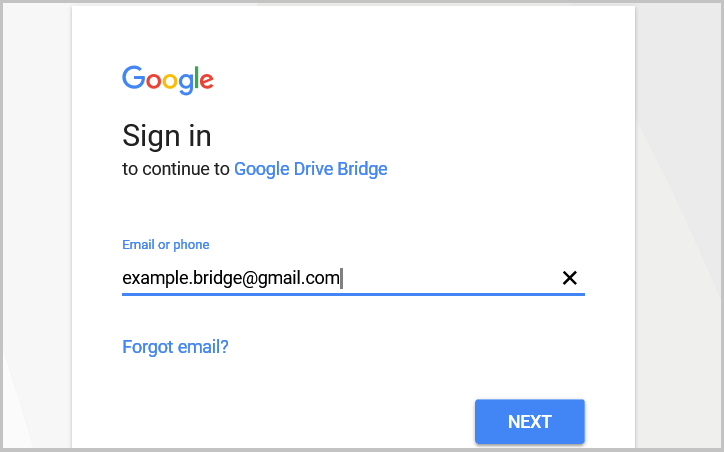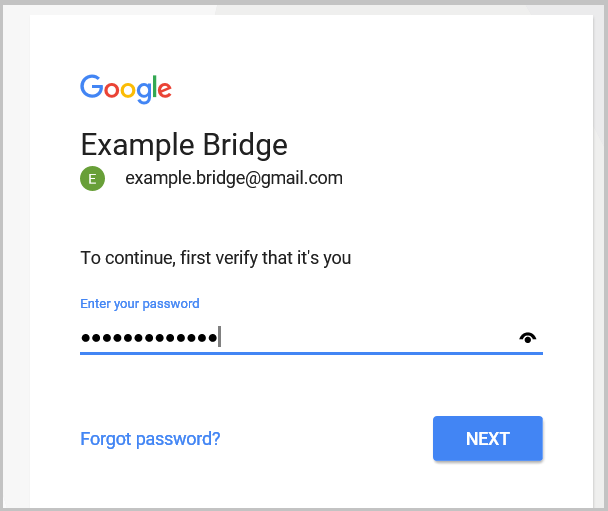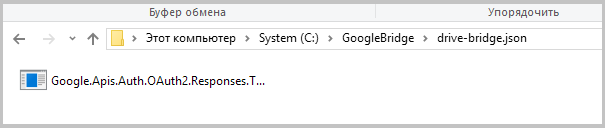### 结论

Provider.mq5 (4.71 KB)
Provider.ex5 (35.89 KB)
User.ex5 (32.23 KB)
User.mq5 (5.5 KB)
CopyObject.mqh (15.53 KB)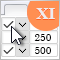图形界面 XI: 表格单元中的文本编辑框和组合框 (统合构建15)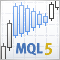旗形形态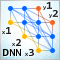深度神经网络 (第 III 部)。样品选择和降维深度神经网络 (第 IV 部)。创建, 训练和测试神经网络模型